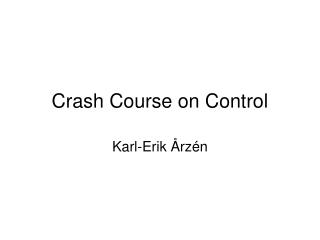DownloadDownload PresentationCrash Course on Control

# Crash Course on Control

Download Presentation## Crash Course on Control

- - - - - - - - - - - - - - - - - - - - - - - - - - - E N D - - - - - - - - - - - - - - - - - - - - - - - - - - -
##### Presentation Transcript

1. Crash Course on Control Karl-Erik Årzén

2. Contents • Introduction • A typical control problem • (Discrete Control Systems) • Continuous Control Systems • Basics • Discrete-Time Implementation • Example: PID Control • Resource-constrained control

3. Dynamic System: • static y = f(u(t)) • dynamic y = f(u(t),u(t-1),…) • State

4. Contents • Introduction • A typical control problem • (Discrete Control Systems) • Continuous Control Systems • Basics • Discrete-Time Implementation • Example: PID Control • Resource-constrained control

5. Discrete Control Systems

6. Contents • Introduction • A typical control problem • (Discrete Control Systems) • Continuous Control Systems • Basics • Discrete-Time Implementation • Example: PID Control • Resource-constrained control

7. Continuous Control: Basic Setting

8. Linear vs NonLinear • The world is nonlinear • Examples: • Nonlinear process dynamics • Nonlinearities is sensors • Nonlinearities in actuators (backlash, friction, ..) • Nonlinearities in implementation platform (quantization)

9. Stability • A key issue for control engineers • Stability of a solution • Stability • Asymptotic stability • For linear systems, stability is a system property • Stability region – left half plane

10. Contents • Introduction • A typical control problem • (Discrete Control Systems) • Continuous Control Systems • Basics • Discrete-Time Implementation • Example: PID Control • Resource-constrained control